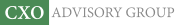Objective research to aid investing decisions

#### Value Investing Strategy (Strategy Overview)

Allocations for October 2020 (Final)
Cash TLT LQD SPY

#### Momentum Investing Strategy (Strategy Overview)

Allocations for October 2020 (Final)
1st ETF 2nd ETF 3rd ETF

# SACEMS Optimization in Depth

| | Posted in: Momentum Investing, Strategic Allocation

The Simple Asset Class ETF Momentum Strategy (SACEMS) each month picks the one, two or three of nine asset class proxies with the highest cumulative total returns over a specified lookback interval. A subscriber proposed instead using the optimal intrinsic (time series or absolute) momentum lookback interval for each asset rather than a common lookback interval for all assets. SACEMS and the proposed approach represent different beliefs (which could both be somewhat true), as follows:

• Many investors adjust asset class allocations with some regularity, such that behaviors of classes are important and coordinated.
• Many investors switch between specific asset classes and cash with some regularity, such that each class may exhibit distinct times series behavior.

To investigate, we consider two ways to measure intrinsic momentum for each asset class proxy:

1. Correlation between next-month return and average monthly return over the past one to 12 months. The lookback interval with the highest correlation has the strongest (linear) relationship between past and future returns and is optimal.
2. Intrinsic momentum, measured as compound annual growth rate (CAGR) for a strategy that is in the asset (cash) when its total return over the past one to 12 months is positive (zero or negative). The lookback interval with the highest CAGR is optimal.

We use the two sets of optimal lookback intervals (optimization-in-depth) to calculate momentum for each asset class proxy as its average monthly return over its optimal lookback interval. We then compare performance statistics for these two alternatives to those for base SACEMS, focusing on: gross CAGR for several intervals; average gross annual return; standard deviation of annual returns; gross annual Sharpe ratio; and, gross maximum drawdown (MaxDD). Using monthly dividend-adjusted prices for SACEMS asset class proxies during February 2006 through September 2019, we find that: